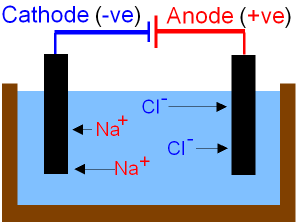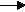gcsescience.com                                                 4                                                 gcsescience.com

Extraction of Metals

Electrolysis of Sodium Chloride.

This page shows the electrolysis of pure sodium chloride.
You get different products
if the sodium chloride is dissolved in water.

Sodium chloride must be heated until it is molten
before it will conduct electricity. Electrolysis
separates the molten ionic compound into its elements.What is a Half Equation?

The reactions at each electrode are called half equations.
The half equations are written so that the
same number of electrons occur in each equation.

2Na+  +  2e-2Na (sodium metal at the (-)cathode).
2Cl-  -  2e-Cl2 (chlorine gas at the (+)anode).

Sodium ions gain electrons (reduction) to form sodium atoms.
Chloride ions lose electrons (oxidation) to form chlorine atoms.
The chlorine atoms combine to form molecules of chlorine gas.

The overall reaction is

2Na+Cl-(l)2Na(s)  +   Cl2(g)

Sodium can be used in street lamps and as a coolant in some
nuclear reactors. See some other examples of electrolysis.

gcsescience.com      The Periodic Table      Index      Metal Quiz      gcsescience.com